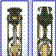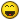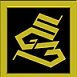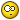Jump to content

# Offset from a reference## Recommended PostsI need to offset a line a certian distance from a construction line in an xref. How do I go about doing this, as I cannot seem to use the offset tool?

##### Share on other sitesDraw a temporary line on top of the construction line and offset it

or...

use Ncopy to copy the line from the xref into the current drawing, then offset.

##### Share on other sitesWe have a lisp that allows this to happen, I will see if I can get a hold of it, as Im not sure if my company will allow me too...

##### Share on other sitesHave you tried to "explode" the line. It may actually be a block unless you are the one who drew it? I have had this problem before as I do not thing a block can be offset with just the offset command.

Just an idea.

##### Share on other sitesI wouldn't try to explode. If it's an x-ref it won't, if it's a block it will, but if you explode it it kind of defeats the purpose of it being a block. Easiest way is probably like lpseifert said, just draw a temporary line on top of it then offset. Since an x-ref is an object in itself, it won't recognize the individual lines within it as lines, and hence won't offset.

##### Share on other sitesHere you go, try this code...Long so had to use 2 posts

;THIS PROGRAM WILL OFFSET XREFERENCED ENTITIES

;LIKE LINE, ARCS AND CIRCLES

;IT WILL ALSO COPY MOST ENTITIES IF YOU CHOOSE A ZERO OFFSET DISTANCE (EVEN TEXT!!!)

;THIS PROGRAM WILL ADJUST FOR SCALE, ROTATION, AND INSERTION POINT OF THE XREF

(DEFUN L-COPY ( ED / TXT A0 CL EL )

(SETQ ED (CDR ED))

(IF (ENTMAKE ED)

(PROGN (SETQ EL (ENTLAST))

(SETQ CL (GETVAR "CLAYER"))

(COMMAND "CHPROP" EL "" "LA" CL "")

);CLOSE IF THEN PROGN

(PROGN (SETQ A0 (ASSOC 0 ED)) (SETQ A0 (CDR A0))

(SETQ TXT (STRCAT "THIS PROGRAM CAN'T HANDLE " A0))

(PRINC TXT)

) ) );CLOSE ELSE PROGN, CLOSE IF ENTMAKE, CLOSE DEFUN L-COPY

;********************************************************************************

(DEFUN L-ARC ( ED DIST PNT / A10 A40 A50 A51 D- )

(SETQ A10 (ASSOC 10 ED)) (SETQ A10 (CDR A10))

(SETQ A40 (ASSOC 40 ED)) (SETQ A40 (CDR A40))

(SETQ A50 (ASSOC 50 ED)) (SETQ A50 (CDR A50))

(SETQ A51 (ASSOC 51 ED)) (SETQ A51 (CDR A51))

(SETQ D- (DISTANCE A10 PNT))

(IF (> D- A40)(SETQ A40 (+ DIST A40))(SETQ A40 (- A40 DIST)));CLOSE IF

(SETQ A50 (POLAR A10 A50 A40)) (SETQ A51 (POLAR A10 A51 A40))

(COMMAND "ARC" "C" A10 A50 A51)

);CLOSE DEFUN L-ARC

;*****************************************************************************

(DEFUN L-CIRCLE ( ED DIST PNT / A10 A40 )

(SETQ A10 (ASSOC 10 ED)) (SETQ A10 (CDR A10))

(SETQ A40 (ASSOC 40 ED)) (SETQ A40 (CDR A40))

(SETQ D- (DISTANCE A10 PNT))

(IF (> D- A40)(SETQ A40 (+ DIST A40))(SETQ A40 (- A40 DIST)));CLOSE IF

(COMMAND "CIRCLE" A10 A40)

);CLOSE DEFUN L-CIRCLE

;*****************************************************************************

(DEFUN L-LINE (ED DIST PNT / A10 A11 ANG1 ANG2 ANG- ANG-ABS )

(SETQ A10 (ASSOC 10 ED)) (SETQ A10 (CDR A10))

(SETQ A11 (ASSOC 11 ED)) (SETQ A11 (CDR A11))

(SETQ ANG1 (ANGLE A10 A11)) (SETQ ANG2 (ANGLE A10 PNT))

(SETQ ANG- (- ANG1 ANG2)) (SETQ ANG-ABS (ABS ANG-))

(COND ((AND (> ANG- 0)(

((AND (> ANG- 0)(> ANG-ABS pi))(SETQ ANG1 (+ ANG1 (/ pi 2))))

((AND (

((AND ( ANG-ABS pi))(SETQ ANG1 (- ANG1 (/ pi 2))))

);CLOSE CONDITION

(SETQ A10 (POLAR A10 ANG1 DIST)) (SETQ A11 (POLAR A11 ANG1 DIST))

(COMMAND "LINE" A10 A11 "")

);CLOSE DEFUN L-LINE

;**************************************************************************

(DEFUN PL-START ( EN / ED A0 A-2 )

(SETQ EN (ENTNEXT EN)) (SETQ ED (ENTGET EN))

(SETQ A0 (ASSOC 0 ED)) (SETQ A0 (CDR A0))

(WHILE (/= A0 "SEQEND")

(SETQ EN (ENTNEXT EN)) (SETQ ED (ENTGET EN))

(SETQ A0 (ASSOC 0 ED)) (SETQ A0 (CDR A0))

);CLOSE WHILE

(SETQ A-2 (ASSOC -2 ED)) (SETQ EN (CDR A-2))

);CLOSE DEFUN PL-START

;**************************************************************************

(DEFUN L-PLINE ( EN DIST PNT / ED A0 EL CL )

(SETQ CL (GETVAR "CLAYER"))

(SETQ EN (PL-START EN)) (SETQ ED (ENTGET EN))

(SETQ A0 (ASSOC 0 ED)) (SETQ A0 (CDR A0))

(WHILE (/= A0 "SEQEND")

(SETQ ED (SUBST (CONS 8 CL) (ASSOC 8 ED) ED))

(SETQ ED (CDR ED)) (ENTMAKE ED)

(SETQ EN (ENTNEXT EN)) (SETQ ED (ENTGET EN))

(SETQ A0 (ASSOC 0 ED)) (SETQ A0 (CDR A0))

);CLOSE WHILE

(SETQ ED (CDR ED))

(IF (ENTMAKE ED) (PROGN

(SETQ EL (ENTLAST))

(IF (/= DIST 0) (PROGN

(COMMAND "OFFSET" DIST EL PNT "")

(COMMAND "ERASE" EL "") (SETQ EL (ENTLAST))

) );CLOSE PROGN, CLOSE IF

);CLOSE PROGN

(PRINC "\nTHIS PROGRAM CAN'T HANDLE THAT ENTITY")

));CLOSE IF, CLOSE DEFUN L-PLINE

;**************************************************************************

(DEFUN MTRXLIST (MTRX / M1 M2 M3 M4 M1X M1Y M1Z M2X M2Y M2Z

M3X M3Y M3Z M4X M4Y M4Z )

(SETQ M1 (NTH 0 MTRX)) (SETQ M2 (NTH 1 MTRX)) (SETQ M3 (NTH 2 MTRX))

(SETQ M4 (NTH 3 MTRX)) (SETQ M1X (NTH 0 M1)) (SETQ M1Y (NTH 1 M1))

(SETQ M1Z (NTH 2 M1)) (SETQ M2X (NTH 0 M2)) (SETQ M2Y (NTH 1 M2))

(SETQ M2Z (NTH 2 M2)) (SETQ M3X (NTH 0 M3)) (SETQ M3Y (NTH 1 M3))

(SETQ M3Z (NTH 2 M3)) (SETQ M4X (NTH 0 M4)) (SETQ M4Y (NTH 1 M4))

(SETQ M4Z (NTH 2 M4))

(SETQ M-LST (LIST M1X M1Y M1Z M2X M2Y M2Z M3X M3Y M3Z M4X M4Y M4Z))

);CLOSE DEFUN MTRXLIST

;**************************************************************************

(DEFUN MTRXCNVT ( PNT ML / PX PY PZ LNG XX YY ZZ )

(SETQ PX (NTH 0 PNT)) (SETQ PY (NTH 1 PNT)) (SETQ LNG (LENGTH PNT))

(IF (= LNG 3)(SETQ PZ (NTH 2 PNT))(SETQ PZ 0));CLOSE IF

(SETQ XX (+ (* PX (NTH 0 ML)) (* PY (NTH 3 ML)) (* PZ (NTH 6 ML)) (NTH 9 ML)))

(SETQ YY (+ (* PX (NTH 1 ML)) (* PY (NTH 4 ML)) (* PZ (NTH 7 ML)) (NTH 10 ML)))

(SETQ ZZ (+ (* PX (NTH 2 ML)) (* PY (NTH 5 ML)) (* PZ (NTH 8 ML)) (NTH 11 ML)))

(SETQ PNT (LIST XX YY ZZ))

);CLOSE DEFUN MTRXCNVT

;**************************************************************************

(DEFUN M-ARC ( ED DIST PNT M-LIST SCL / A10 A40 A50 A51 D- )

(princ ed)

(SETQ A10 (ASSOC 10 ED)) (SETQ A10 (CDR A10))

(SETQ A40 (ASSOC 40 ED)) (SETQ A40 (CDR A40))(SETQ A40 (* A40 SCL))

(SETQ A50 (ASSOC 50 ED)) (SETQ A50 (CDR A50))

(SETQ A51 (ASSOC 51 ED)) (SETQ A51 (CDR A51))

(SETQ A50 (POLAR A10 A50 A40)) (SETQ A51 (POLAR A10 A51 A40))

(SETQ A10 (MTRXCNVT A10 M-LIST)) (SETQ A50 (MTRXCNVT A50 M-LIST))

(SETQ A51 (MTRXCNVT A51 M-LIST)) (SETQ A50 (ANGLE A10 A50))

(SETQ A51 (ANGLE A10 A51)) (SETQ D- (DISTANCE A10 PNT))

(IF (> D- A40)(SETQ A40 (+ DIST A40))(SETQ A40 (- A40 DIST)));CLOSE IF

(SETQ A50 (POLAR A10 A50 A40)) (SETQ A51 (POLAR A10 A51 A40))

(IF (OR (AND ( (NTH 4 M-LIST) 0))

(AND ( (NTH 0 M-LIST) 0)))

(COMMAND "ARC" "C" A10 A51 A50)

(COMMAND "ARC" "C" A10 A50 A51)

);CLOSE IF

);CLOSE DEFUN M-ARC

;**************************************************************************

(DEFUN M-CIRCLE ( ED DIST PNT M-LIST SCL / A10 A40 )

(SETQ A10 (ASSOC 10 ED)) (SETQ A10 (CDR A10))

(SETQ A40 (ASSOC 40 ED)) (SETQ A40 (CDR A40)) (SETQ A40 (* A40 SCL))

(SETQ A10 (MTRXCNVT A10 M-LIST)) (SETQ D- (DISTANCE A10 PNT))

(IF (> D- A40)(SETQ A40 (+ DIST A40))(SETQ A40 (- A40 DIST)));CLOSE IF

(COMMAND "CIRCLE" A10 A40)

);CLOSE DEFUN M-CIRCLE

;**************************************************************************

(DEFUN M-LINE ( ED DIST PNT M-LIST / A10 A11 ANG1 ANG2 ANG-ABS )

(SETQ A10 (ASSOC 10 ED)) (SETQ A10 (CDR A10))

(SETQ A11 (ASSOC 11 ED)) (SETQ A11 (CDR A11))

(SETQ A10 (MTRXCNVT A10 M-LIST)) (SETQ A11 (MTRXCNVT A11 M-LIST))

(SETQ ANG1 (ANGLE A10 A11)) (SETQ ANG2 (ANGLE A10 PNT))

(SETQ ANG- (- ANG1 ANG2)) (SETQ ANG-ABS (ABS ANG-))

(COND ((AND (> ANG- 0)(

((AND (> ANG- 0)(> ANG-ABS pi))(SETQ ANG1 (+ ANG1 (/ pi 2))))

((AND (

((AND ( ANG-ABS pi))(SETQ ANG1 (- ANG1 (/ pi 2))))

);CLOSE CONDITION

(SETQ A10 (POLAR A10 ANG1 DIST)) (SETQ A11 (POLAR A11 ANG1 DIST))

(COMMAND "LINE" A10 A11 "")

);CLOSE DEFUN M-LINE

##### Share on other sites```
;**************************************************************************
(DEFUN M-PLINE ( EN DIST PNT M-LIST / ED A0 A10 ZZ EL CL )
(SETQ EN (PL-START EN)) (SETQ ED (ENTGET EN))
(SETQ CL (GETVAR "CLAYER"))
(SETQ A0 (ASSOC 0 ED)) (SETQ A0 (CDR A0))
(SETQ A10 (ASSOC 10 ED)) (SETQ A10 (CDR A10))
(SETQ A10 (MTRXCNVT A10 M-LIST)) (SETQ ZZ (NTH 2 A10))
(SETQ A10 (LIST 0 0 ZZ))
(SETQ ED (SUBST (CONS 8 CL) (ASSOC 8 ED) ED))
(SETQ ED (SUBST (CONS 10 A10) (ASSOC 10 ED) ED))
(SETQ ED (CDR ED)) (ENTMAKE ED) (SETQ EN (ENTNEXT EN))
(SETQ ED (ENTGET EN)) (SETQ A0 (ASSOC 0 ED)) (SETQ A0 (CDR A0))
(WHILE (/= A0 "SEQEND")
(SETQ A10 (ASSOC 10 ED)) (SETQ A10 (CDR A10))
(SETQ A10 (MTRXCNVT A10 M-LIST))
(SETQ ED (SUBST (CONS 8 CL) (ASSOC 8 ED) ED))
(SETQ ED (SUBST (CONS 10 A10) (ASSOC 10 ED) ED))
(SETQ ED (CDR ED)) (ENTMAKE ED) (SETQ EN (ENTNEXT EN))
(SETQ ED (ENTGET EN)) (SETQ A0 (ASSOC 0 ED)) (SETQ A0 (CDR A0))
);CLOSE WHILE
(SETQ ED (CDR ED))
(IF (ENTMAKE ED) (PROGN
(SETQ EL (ENTLAST))
(IF (/= DIST 0) (PROGN
(COMMAND "OFFSET" DIST EL PNT "") (COMMAND "ERASE" EL "")
(SETQ EL (ENTLAST))
) );CLOSE PROGN, CLOSE IF
);CLOSE PROGN
(PRINC "\nTHIS PROGRAM CAN'T HANDLE THAT ENTITY")
));CLOSE IF, CLOSE DEFUN M-PLINE
;************************************************************************
(DEFUN M-COPY ( ED M-LIST / TXT A0 CL EL A10 A8 )
(SETQ A50 (ASSOC 50 ED)) (SETQ A50 (CDR A50))
(SETQ A10 (ASSOC 10 ED)) (SETQ A10 (CDR A10))
(SETQ A-PNT (POLAR A10 A50 5))
(SETQ A10 (MTRXCNVT A10 M-LIST))
(SETQ A-PNT (MTRXCNVT A-PNT M-LIST))
(SETQ ED (SUBST (CONS 10 A10) (ASSOC 10 ED) ED))
(SETQ M-ANG (ANGLE A10 A-PNT))
(SETQ ED (SUBST (CONS 50 M-ANG) (ASSOC 50 ED) ED))
(IF (ASSOC 11 ED) (PROGN
(SETQ A11 (ASSOC 11 ED)) (SETQ A11 (CDR A11))
(SETQ A11 (MTRXCNVT A11 M-LIST))
(SETQ ED (SUBST (CONS 11 A11) (ASSOC 11 ED) ED))
))CLOSE PROGN, CLOSE IF
(IF (ASSOC 12 ED) (PROGN
(SETQ A12 (ASSOC 12 ED)) (SETQ A12 (CDR A12))
(SETQ A12 (MTRXCNVT A12 M-LIST))
(SETQ ED (SUBST (CONS 12 A12) (ASSOC 12 ED) ED))
))CLOSE PROGN, CLOSE IF
(IF (ASSOC 13 ED) (PROGN
(SETQ A13 (ASSOC 13 ED)) (SETQ A13 (CDR A13))
(SETQ A13 (MTRXCNVT A13 M-LIST))
(SETQ ED (SUBST (CONS 13 A13) (ASSOC 13 ED) ED))
))CLOSE PROGN, CLOSE IF
(IF (ASSOC 14 ED) (PROGN
(SETQ A14 (ASSOC 14 ED)) (SETQ A14 (CDR A14))
(SETQ A14 (MTRXCNVT A14 M-LIST))
(SETQ ED (SUBST (CONS 14 A14) (ASSOC 14 ED) ED))
))CLOSE PROGN, CLOSE IF
(IF (ASSOC 15 ED) (PROGN
(SETQ A15 (ASSOC 15 ED)) (SETQ A15 (CDR A15))
(SETQ A15 (MTRXCNVT A15 M-LIST))
(SETQ ED (SUBST (CONS 15 A15) (ASSOC 15 ED) ED))
))CLOSE PROGN, CLOSE IF
(IF (ASSOC 16 ED) (PROGN
(SETQ A16 (ASSOC 16 ED)) (SETQ A16 (CDR A16))
(SETQ A16 (MTRXCNVT A16 M-LIST))
(SETQ ED (SUBST (CONS 16 A16) (ASSOC 16 ED) ED))
))CLOSE PROGN, CLOSE IF
(IF (ASSOC 17 ED) (PROGN
(SETQ A17 (ASSOC 17 ED)) (SETQ A17 (CDR A17))
(SETQ A17 (MTRXCNVT A17 M-LIST))
(SETQ ED (SUBST (CONS 17 A17) (ASSOC 17 ED) ED))
))CLOSE PROGN, CLOSE IF
(IF (ASSOC 18 ED) (PROGN
(SETQ A18 (ASSOC 18 ED)) (SETQ A18 (CDR A18))
(SETQ A18 (MTRXCNVT A18 M-LIST))
(SETQ ED (SUBST (CONS 18 A18) (ASSOC 18 ED) ED))
))CLOSE PROGN, CLOSE IF
(SETQ CL (GETVAR "CLAYER"))
(SETQ ED (SUBST (CONS 8 CL) (ASSOC 8 ED) ED))
(PRINC ED)
(SETQ ED (CDR ED))
(IF (NOT (ENTMAKE ED)) (PROGN
(SETQ A0 (ASSOC 0 ED))
(SETQ A0 (CDR A0))
(SETQ TXT (STRCAT "THIS PROGRAM CAN'T HANDLE " A0))
(PRINC TXT)
) ) );CLOSE PROGN, CLOSE IF ENTMAKE, CLOSE DEFUN M-COPY
;**************************************************************************
(DEFUN SCALE-IT ( EL / LNG CNTR EN ED A41 SCL )
(SETQ LNG (LENGTH EL)) (SETQ CNTR 0) (SETQ SCL 1)
(WHILE (< CNTR LNG)
(SETQ EN (CAR EL)) (SETQ EL (CDR EL)) (SETQ ED (ENTGET EN))
(IF (ASSOC 41 ED) (PROGN
(SETQ A41 (ASSOC 41 ED)) (SETQ A41 (CDR A41))
(SETQ SCL (* SCL A41))
));CLOSE PROGN, CLOSE IF
(SETQ CNTR (+ CNTR 1))
);CLOSE WHILE
(SETQ SCL (ABS SCL))
);CLOSE DEFUN
(defun SaveAndSetSystemVariables (lst / )
(setq *SavedSystemVariables* (mapcar '(lambda (lstV) (cons (car lstV) (getvar (car lstV)))) lst))
(SetSystemVariables lst)
); defun
(defun RestoreSystemVariables (/)
(SetSystemVariables *SavedSystemVariables*)
(setq *SavedSystemVariables* nil)
); defun
(defun SetSystemVariables (lst /)
(mapcar '(lambda (lstV) (setvar (car lstV) (cdr lstV))) lst)
); defun
(defun off:initialize ( / )
(setq olderror *error*
*error* off:error
); setq
(SaveAndSetSystemVariables (list (cons "OSMODE" 0)
(cons "ORTHOMODE" 0)
(cons "CMDECHO" 0)
); list
); SaveAndSetSystemVariables
); defun
(defun off:finalize ( / )
(RestoreSystemVariables)
(setq *error* olderror)
); defun

;**************************************************************************
(DEFUN C:OFF ( / off:error olderror) ;T1 CHK E OFF-PNT EN ED A0 E-LNG MTRX M-LIST ENS E-SCL )
(defun off:error (msg / )
(if
(or
(= msg "Function cancelled")
(= msg "quit / exit abort")
); or
nil
(prompt (strcat "\nError: " msg))
); if
(off:finalize)
); defun
(off:initialize)
(IF (NOT DIST-OFF) (PROGN
(INITGET 1)
(SETQ DIST-OFF (GETDIST "\nENTER THE OFFSET DISTANCE OR <0> TO COPY:> "))
);CLOSE THEN PROGN
(PROGN
(SETQ T1 (RTOS DIST-OFF 2 2))
(SETQ T1 (STRCAT "\nENTER THE OFFSET DISTANCE OF <RETURN> FOR " T1 " :> "))
(PRINC T1) (SETQ CHK (GETDIST)) (IF CHK (SETQ DIST-OFF CHK))
) );CLOSE ELSE PROGN, CLOSE IF
(IF (/= DIST-OFF 0) (SETQ E (NENTSEL "\nPICK ITEM TO OFFSET"))
(SETQ E (NENTSEL "\nPICK ITEM TO COPY"))
);CLOSE IF
(PRINC "\nTHIS IS E")
(PRINC E)
(PRINC "\nTHIS IS E")
(WHILE E
(IF (/= DIST-OFF 0)
(SETQ OFF-PNT (GETPOINT "\nPICK SIDE TO OFFSET"))
(SETQ OFF-PNT (LIST 0 0 0))
);CLOSE IF
(SETQ EN (CAR E)) (SETQ ED (ENTGET EN))
(SETQ A0 (ASSOC 0 ED)) (SETQ A0 (CDR A0))
(SETQ E-LNG (LENGTH E))
(IF (= E-LNG 2) (COND
((= A0 "ARC") (L-ARC ED DIST-OFF OFF-PNT))
((= A0 "CIRCLE") (L-CIRCLE ED DIST-OFF OFF-PNT))
((= A0 "LINE") (L-LINE ED DIST-OFF OFF-PNT))
((= A0 "VERTEX")(L-PLINE EN DIST-OFF OFF-PNT))
((= DIST-OFF 0) (L-COPY ED))
((/= DIST-OFF 0) (PRINC "\nTHIS PROGRAM CANT HANDLE THAT"))
);CLOSE IF THEN CONDITION
(PROGN
(SETQ MTRX (NTH 2 E)) (SETQ M-LIST (MTRXLIST MTRX))
(SETQ ENS (LAST E))
(SETQ E-SCL (SCALE-IT ENS))
(COND
((= A0 "ARC") (M-ARC ED DIST-OFF OFF-PNT M-LIST E-SCL))
((= A0 "CIRCLE") (M-CIRCLE ED DIST-OFF OFF-PNT M-LIST E-SCL))
((= A0 "LINE") (M-LINE ED DIST-OFF OFF-PNT M-LIST))
((= A0 "VERTEX") (M-PLINE EN DIST-OFF OFF-PNT M-LIST))
((= DIST-OFF 0) (M-COPY ED M-LIST))
((/= DIST-OFF 0) (PRINCE "\nTHIS PROGRAM CANT HANDLE THAT"))
) ) );CLOSE CONDITION, CLOSE PROGN, CLOSE IF
(IF (/= DIST-OFF 0) (SETQ E (NENTSEL "\nPICK NEXT ITEM TO OFFSET:> "))
(SETQ E (NENTSEL "\nPICK NEXT ITEM TO COPY:> "))
) )
(off:finalize)
(princ)
);CLOSE IF, CLOSE WHILE E, CLOSE DEFUN OFF2
;*************************************************************************
```

##### Share on other sitesLONG?!? more like a novel smorales02 LOL,Sorry no offense bad joke on my part.##### Share on other sitesI do not take responsibility for the above code...

##### Share on other sitesas i say i do appoloise i tired an get a very warped sense of humour when that happens. (11:10pm over the pond)

back to the thread now,

from what little bit of lisp i know, i'd say it looks good.

##### Share on other sites
• 2 weeks later...omg

Just get them to bind the exref, and re-send

##### Share on other sitesBinding the x-ref kind of defeats the purpose of it being an x-ref though. I suppose it depends on the complexity of the x-ref and how much binding it would complicate the drawing.

##### Share on other sitesomg

Just get them to bind the exref, and re-send

How does binding the xref contribute to offseting a line in the offset?Maybe you could just open the xref in place, copy w/ basepoint all lines you plan to offset, close the xref then paste copied line on same base point.

##### Share on other sitesYa'know I've actually tried to do that before and AutoCAD would't let me basepoint copy when I'm working on an x-ref within another drawing. I'd have to actually open the x-ref file itself, then do a basepoint copy, then paste it back in the first drawing. Perhaps that's just my older version of AutoCAD though, maybe the newer ones let you do that.

##### Share on other sitesHow does binding the xref contribute to offseting a line in the offset?

It does not, just gives you ability to use that xref. Has to be bound from the sender

## Join the conversation

You can post now and register later. If you have an account, sign in now to post with your account.
Note: Your post will require moderator approval before it will be visible.Reply to this topic...

×   Pasted as rich text.   Restore formatting

Only 75 emoji are allowed.

×   Your link has been automatically embedded.   Display as a link instead

×   Your previous content has been restored.   Clear editor

×   You cannot paste images directly. Upload or insert images from URL.

×
×
×
• Create New...